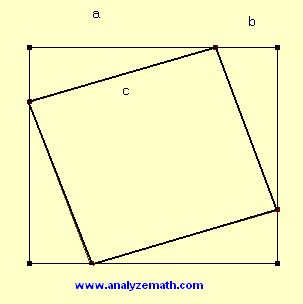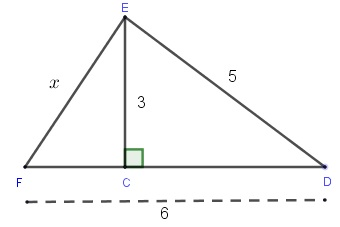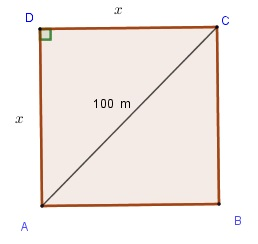# Pythagorean Theorem and Problems with Solutions

Explore some simple proofs of the Pythagorean theorem and its converse and use them to solve problems. Detailed solutions to the problems are also presented.

## Proofs Of Pythagorean Theorem

### Proof 1

In the figure below are shown two squares whose sides are a + b and c. let us write that the area of the large square is the area of the small square plus the total area of all 4 congruent right triangles in the corners of the large square.(a + b)
2 = c 2 + 4 (1 / 2) (a b)
Expand the left hand side of the above equality, and simplify the last term on the right
a
2 + b 2 + 2 a b = c 2 + 2 a b
Simplify to obtain
a 2 + b 2 = c 2
a and b are the sides of the right triangle and c is its hypotenuse.

### Proof 2

We first start with a right triangle and then complete it to make a rectangle as shown in the figure below which in turn in made up of three triangles.The fact that the sides of the rectangle are parallel, that gives rise to angles being congruent (equal in size) in all three triangles shown in the figure which leads to the triangles being similar. We first consider triangles ABE and AED which are similar because of the equality of angles. The proportionality of corresponding sides (a in triangle ABE corresponds to c in triangle AED since both faces a right angle, x in triangle ABE corresponds to a in triangle AED both faces congruent (equal) angles) gives
a / c = x / a which may be written as a
2 = c x
We next consider triangles ECD and AED which are similar because of the equality of angles. The corresponding sides are proportional, hence
b / c = y / b which may be written as b
2 = c y
We now add the sides of the equalities a
2 = c x and b 2 = c y to obtain
a
2 + b 2 = c x + c y = c (x + y)
Use the fact that c = x + y to write
a
2 + b 2 = c 2

### Converse of the Pythagorean Theorem

The converse of the Pythagorean theorem sates that: if a, b and c are the lengths of a triangle with c the longest side and a 2 + b 2 = c 2 then this triangle is a right triangle and c is the length of its hypotenuse.

## Pythagorean Theorem and Triangle Problems

Problem 1
Find the area of a right triangle whose hypotenuse is equal to 10 cm and one of its sides is 6 cm.

Problem 2
Which of the following may be the lengths of the sides of a right angled triangle?
a) (2 , 3 , 4)    b) (12 , 16 , 20)    c) (3√2 , 3√2 , 6)

Problem 3
The points A(0 , 2), B(-2 , -1) and C(- 1 , k) are the vertices of a right triangle with hypotenuse AB. Find k.

Problem 4
One side of a triangle has a length that is 2 meters less that its hypotenuse and its second side has a length that is 4 meters less that its hypotenuse. Find the perimeter of the triangle.

Problem 5
Find the perimeter of an equilateral triangle whose height is 60 cm.

Problem 6
Calculate the are of a square field whose diagonal is 100 meters.

Problem 7
Find x in the two right triangles figure below.## Detailed Solutions to the Above Problems

Solution to Problem 1
Given the hypotenuse and one of the sides, we use the Pythagorean theorem to find the second side x as followsx
2 + 6 2 = 10 2
Solve for x
x = √ (10
2 - 6 2) = 8
Area of the triangle = (1 / 2) height � base
The two sides of a right triangle make a right angle and may therefore be considered as the height and the base. Hence
Area of the triangle = (1 / 2) 6 � 8 = 24 cm
2

Solution to Problem 2
We use the converse of the Pythagorean theorem to solve this problem.
a) (2 , 3 , 4) : 4 is the length of the longest side
2
2 + 3 2 = 13
4
2 = 16
since 2
2 + 3 2 is NOT EQUAL to 4 2, (2 , 3 , 4) are not the lengths of the sides of a right triangle.

b) (12 , 16 , 20) : 20 is the longest side
12
2 + 16 2 = 400
20
2 = 400
since 12
2 + 16 2 is EQUAL to 20 2, (12 , 16 , 20) are the lengths of the sides of a right triangle.

c) (3√2 , 3√2 , 6) : 6 is the longest side
(3√2)
2 + (3√2) 2 = 36
6
2 = 36
(3√2)
2 + (3√2) 2 is EQUAL to 6 2, therefore (3√2 , 3√2 , 6) are the length of a right triangle with the length of the hypotenuse equal to 6. Also since two sides have equal length to 3√2, the right triangle is isosceles.

Solution to Problem 3
The points A(0 , 2), B(-2 , -1) and C(- 1 , k) are the vertices of a right triangle with hypotenuse AB. Find k.
Use the formula to find the distance squared between two points given by d
2 = (x 2 - x1) 2 + (y 2 - y1) 2 to find the square of the length of the hypotenuse AB and the sides AC and BC.
AB
2 = (-2 - 0) 2 + (-1 - 2) 2 = 13
AC
2 = (-1 - 0) 2 + (k - 2) 2 = k 2 - 4 k + 5
BC
2 = (-1 - (-2)) 2 + (k - (-1)) 2 = k 2 +2 k + 2
AB is the hypotenuse of the triangle, we now use the Pythagorean theorem AB
2 = AC 2 + BC 2 to obtain an equation in k.
13 = k
2 - 4 k + 5 + k 2 + 2 k + 2
2 k
2 - 2 k - 6 = 0
k
2 - k - 3 = 0
Solve for k the above quadratic equation to obtain two solutions k
1 and k 2 given by
k
1 = ( 1 + √ (13) ) / 2 ≈ 2.30 and k 2 = ( 1 - √ (13) ) / 2 ≈ - 1.30
There are two possible points C
1 and C2 that make a right triangle with A and B and whose coordinates are given by:
C
1 (- 1 , 2.30) and C2 (- 1 , - 1.30)
The graphical interpretation of the solution to this problem is shown below. Solution k
1 corresponds to the "red" right triangle and solution k 2 corresponds to the "blue" right triangle.Solution to Problem 4
Let x be the hypotenuse of the right triangle. One side is x - 2 and the other is x - 4 as shown below. We need to find in order to find that sides and then the perimeter.Use the Pythagorean to write
x
2 = (x - 2) 2 + (x - 4) 2
Expand and group to obtain the quadratic equation
x
2 - 12 x + 20 = 0
Solve to find two solutions
x = 2 and x = 10
Calculate the sides and perimeter for each solution
hypotenuse: x = 2 , side 1 : x - 2 = 0 and side 2 : x - 4 = - 2
The sides of a triangle cannot be zero or negative and therefore x = 2 is not a solution to the given problem.
hypotenuse: x = 10 , side 1 : x - 2 = 8 and side 2 : x - 4 = 6
Perimeter = hypotenuse + side 1 + side 2 = 10 + 8 + 6 = 24 units.

Solution to Problem 5
An equilateral triangle with side x is shown below with height CH = 60 cm. Since it is an equilateral the height CH split the base (segment) AB into two equal segments of size x / 2.We use the Pythagorean theorem on triangle CHB (or CHA) to write
x
2 = 60 2 + ( x / 2) 2
Expand the above equation and rewrite as
3 x
2 / 4 = 3600
Solve for x and take the positive solution since x is the size of the side of the triangle and must be positive.
x = 40 √3 cm
Perimeter = 3 x = 120 √3 cm

Solution to Problem 6
A square of side x and diagonal 100 m is shown below.
Area of the square = x
2
Use the Pythagorean theorem on the triangle ACD to write
x
2 + x 2 = 100 2
Solve for x
2
Area of square = x
2 = 5000 m 2Solution to Problem 7
We first use the Pythagorean theorem to the right triangle ECD in order to find the length of CD
5
2 = 3 2 + CD 2
Solve for CD
CD = √(25 - 9) = 4
We also know that
FC + CD = 6
Hence
FC = 6 - CD = 2
We now use the Pythagorean theorem to the right triangle EFC to write
x
2 = 3 2 + FC 2 = 9 + 4 = 13
Solve for
x = √(13)